# Decimal to Fraction Calculator

## Quick conversion of any decimal to fraction.The decimal-to-fraction calculator is just one of many tools available on Calcopolis for converting decimal numbers to fractions or mixed numbers. It can also help you in converting negative decimals or repeating decimals.

Decimals and fractions are two distinct representations of numbers less than one or between integers. They're both incredibly useful approaches for dealing with the calculations for these specific numbers.

Yet, in some cases, using fractions is more practical than using decimals. You see, a fraction clearly expresses the relationship between two integers in the form of a ratio.

btw. You may be interested also in our ratio simplifier, which can turn complex ratios into simple and more readable versions representing the same mathematical proportion.

Whereas decimals can have the exact numerical value, they lack the clear demonstration that fractions have.

To clarify, assume we have a cake that's divided into 10 slices. If two slices were eaten, it would be easier to describe the remaining portion as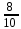rather than 0.8, wouldn't it?

## How to Convert Decimals to Fractions

There are two methods for converting decimals to fractions, depending on the type of decimal.

The first method is for converting rational decimal numbers, which are numbers that can be written as fractions. The second method is for converting repeating decimals, as well as irrational numbers, which are numbers that can't be expressed as fractions.

### 1st Method

#### Step 1: Create a Fraction

Simply create a fraction with the decimal 0.x as the numerator and the number 1 as the denominator to get a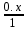#### Step 2: Eliminate the Decimal Places

Count the number of decimal places to the right of the decimal point. The number you discovered y will be the power of the numerator and denominator in a fraction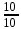. Then, multiply this fraction by the initial fraction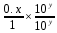, which should remove the decimal places.

#### Step 3: Simplify the Fraction

The first step in simplifying a fraction is to determine if there's a number that can be divided by both the numerator and denominator. After you've identified the Greatest Common Factor (GCF), divide the numerator and denominator by it to get a much simpler fraction!

##### Example: Convert 0.25 to a Fraction
1. First, start by converting 0.25 to a fraction: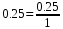2. Then, since there are two places after the decimal we'll multiply the fraction by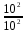: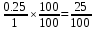3. Finally, to simplify this fraction, multiply it by its GCF, which is 25: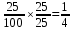### 2nd Method

#### Step 1: Make a Fraction

If you have a repeating decimal or an irrational number, identify the repeating digit and use it as the numerator of a fraction. The number 9 will be repeated with the number of repeated digits in the denominator of this fraction.

For instance, if the repeating decimal is 0.777...., the fraction will be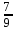. Because the number 7 is the only number that's repeated, there's only one 9. However, if you have 0.363636..., the fraction will be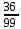. Because there are two numbers 3 and 6, there'll be two 9s.

#### Step 2 (Optional): Add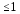Numbers to the Fraction

If the number to the left of the decimal point is greater than or equal to one, such as 1.838383..., it should be added to the fraction. In the end, remember to simplify the fraction you'll get, if possible.

##### Example: Convert 1.6666 to a Fraction
1. First, identify the repeating digit(s) to create a fraction accordingly: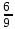2. Then perform addition since the number to the left of the decimal point equals 1:

## When is it helpful to convert a decimal into a fraction?

Converting a decimal into a fraction can be helpful in several situations:

1. Expressing exact values:
Some numbers cannot be expressed as decimals with a finite number of digits but can be represented precisely as fractions. For example, 1/3 cannot be expressed exactly as a decimal but can be expressed exactly as a fraction. In cases where exact values are essential, converting decimals to fractions can be helpful.
2. Working with repeating decimals:
Repeating decimals (such as 0.3333...) can be converted to fractions using a specific formula. Converting repeating decimals to fractions can be helpful in certain situations, such as when working with measurements expressed as repeating decimals (e.g., 1/3 cup).
3. Simplifying calculations:
In some cases, working with fractions can simplify calculations. For example, when multiplying or dividing fractions, it's often easier to convert decimals to fractions first.
4. Working with ratios:
Ratios are often expressed as fractions, so converting decimals to fractions can be helpful when working with ratios.
5. Converting between units:
Sometimes, it may be necessary to convert between units expressed as fractions (such as inches or feet) and units expressed as decimals (such as meters or centimeters). In such cases, converting decimals to fractions can make the conversion process more manageable.
6. Working with mixed numbers:
Mixed numbers are a combination of a whole number and a fraction. Converting decimals to mixed numbers can be helpful in certain situations, such as when working with measurements that are expressed as mixed numbers (e.g., 1 1/2 cup of flour).
7. Solving equations:
In some cases, it may be easier to solve an equation by converting decimals to fractions first. For example, if an equation involves adding or subtracting decimals, it may be simpler to convert them to fractions before calculating.
8. Drawing charts:
When drawing a chart, it may be easier to identify key points (such as intercepts or critical points) if the function is expressed as a fraction instead of a decimal.
9. Representing probabilities:
Probabilities are often expressed as decimals (e.g., 0.25) but can also be expressed as fractions. For example, suppose you are playing a game with 20% of winning, which is a 1 in 5 chance or 1/5.

## Alternatives

Instead of fractions, decimals may be presented as percentage values or ratios, depending on your needs. Percentages are sometimes more convenient, but people often make mistakes in percentage operations.

If you wish to avoid them, use our percent calculator and percent to fraction calculator.

In order to revert this operation, use our dedicated tool and reverse fractions to decimals

### Authors

Created by Lucas Krysiak on 2022-12-21 16:53:53 | Last review by Mike Kozminsky on 2022-12-21 13:09:43